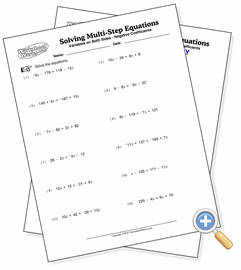# Variable on Both Sides

## Solving Equations With Like TermsSolve equations involving like terms across the equals sign

These equations are solved by combining like terms, followed by the same logic as two-step equations. Terms with the variable will be on both sides of the equation. All problems resolve to integers. You can adjust the difficulty of the problems by increasing the values size, using negative coefficients and flipping the sides of the equations.